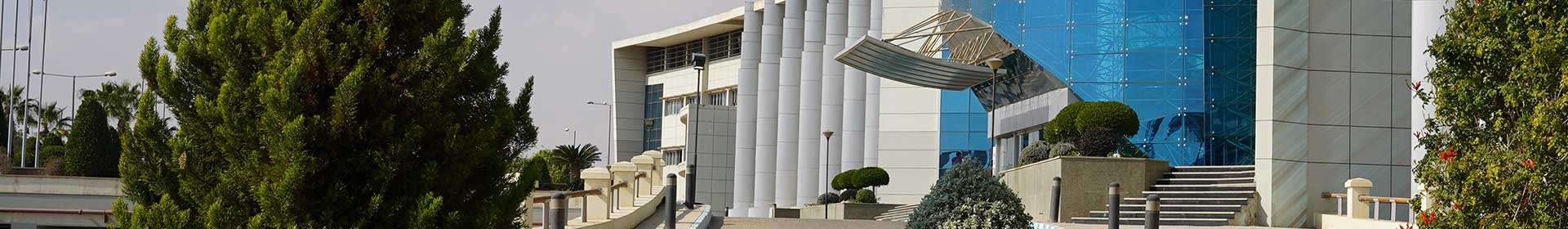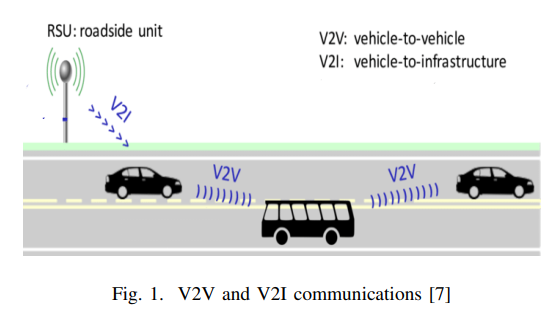Conference Paper

# Collision Probability Computation for Road Intersections Based on Vehicle to Infrastructure Communication

By
Shawki M.
Saeed Darweesh M.

In recent years, many probability models proposed to calculate the collision probability for each vehicle and those models used in collision avoidance algorithms and intersection management algorithms. In this paper, we introduce a method to calculate the collision probability of vehicles at an urban intersection. The proposed model uses the current position, speed, acceleration, and turning direction then each vehicle shares its required information to the roadside unit (RSU) via the Vehicle to Infrastructures (V2I). RSU can predict each vehicle's path in intersections by using the received data. By considering vehicle dimensions in our calculation, RSU will detect a possible collision point and time to collision (TTC) for moving vehicles at the intersection. Simulation results show that this model can detect collisions occurrence early, so it will decrease the probability of a collision occurs. © 2020 IEEE.